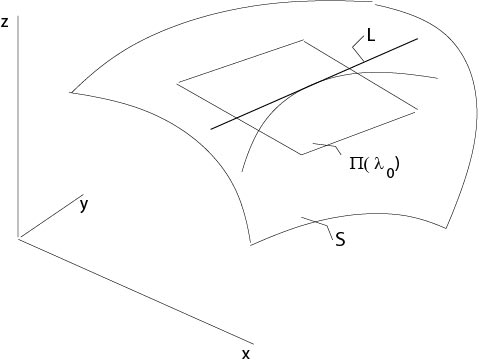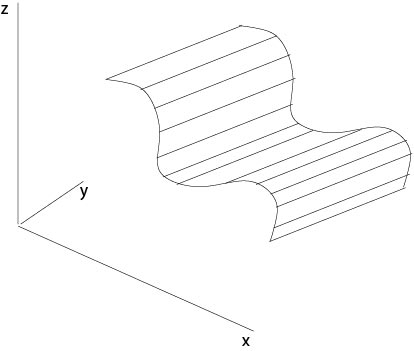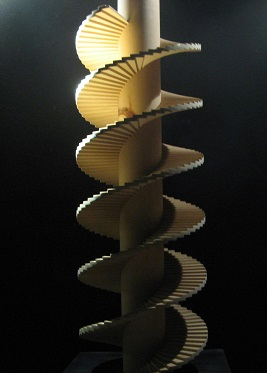$$\newcommand{\id}{\mathrm{id}}$$ $$\newcommand{\Span}{\mathrm{span}}$$ $$\newcommand{\kernel}{\mathrm{null}\,}$$ $$\newcommand{\range}{\mathrm{range}\,}$$ $$\newcommand{\RealPart}{\mathrm{Re}}$$ $$\newcommand{\ImaginaryPart}{\mathrm{Im}}$$ $$\newcommand{\Argument}{\mathrm{Arg}}$$ $$\newcommand{\norm}{\| #1 \|}$$ $$\newcommand{\inner}{\langle #1, #2 \rangle}$$ $$\newcommand{\Span}{\mathrm{span}}$$

# 2.1: Linear Equations

$$\newcommand{\vecs}{\overset { \rightharpoonup} {\mathbf{#1}} }$$ $$\newcommand{\vecd}{\overset{-\!-\!\rightharpoonup}{\vphantom{a}\smash {#1}}}$$$$\newcommand{\id}{\mathrm{id}}$$ $$\newcommand{\Span}{\mathrm{span}}$$ $$\newcommand{\kernel}{\mathrm{null}\,}$$ $$\newcommand{\range}{\mathrm{range}\,}$$ $$\newcommand{\RealPart}{\mathrm{Re}}$$ $$\newcommand{\ImaginaryPart}{\mathrm{Im}}$$ $$\newcommand{\Argument}{\mathrm{Arg}}$$ $$\newcommand{\norm}{\| #1 \|}$$ $$\newcommand{\inner}{\langle #1, #2 \rangle}$$ $$\newcommand{\Span}{\mathrm{span}}$$ $$\newcommand{\id}{\mathrm{id}}$$ $$\newcommand{\Span}{\mathrm{span}}$$ $$\newcommand{\kernel}{\mathrm{null}\,}$$ $$\newcommand{\range}{\mathrm{range}\,}$$ $$\newcommand{\RealPart}{\mathrm{Re}}$$ $$\newcommand{\ImaginaryPart}{\mathrm{Im}}$$ $$\newcommand{\Argument}{\mathrm{Arg}}$$ $$\newcommand{\norm}{\| #1 \|}$$ $$\newcommand{\inner}{\langle #1, #2 \rangle}$$ $$\newcommand{\Span}{\mathrm{span}}$$

Let us begin with the linear homogeneous equation
\begin{equation}
\label{homtwo}
a_1(x,y)u_x+a_2(x,y)u_y=0.
\end{equation}
Assume there is a $$C^1$$-solution $$z=u(x,y)$$. This function defines a surface $$S$$ which has at $$P=(x,y,u(x,y))$$ the normal
$${\bf N}=\frac{1}{\sqrt{1+|\nabla u|^2}}(-u_x,-u_y,1)$$
and the tangential plane defined by
$$\zeta-z=u_x(x,y)(\xi-x)+u_y(x,y)(\eta-y).$$
Set $$p=u_x(x,y)$$, $$q=u_y(x,y)$$ and $$z=u(x,y)$$. The tuple $$(x,y,z,p,q)$$ is called surface element and the tuple $$(x,y,z)$$ support of the surface element. The tangential plane is defined by the surface element. On the other hand, differential equation (\ref{homtwo})
$$a_1(x,y)p+a_2(x,y)q=0$$
defines at each support $$(x,y,z)$$ a bundle of planes if we consider all $$(p,q)$$ satisfying this equation. For fixed $$(x,y)$$, this family of planes $$\Pi(\lambda)=\Pi(\lambda;x,y)$$ is defined by a one parameter family of ascents $$p(\lambda)=p(\lambda;x,y)$$, $$q(\lambda)=q(\lambda;x,y)$$.
The envelope of these planes is a line since
$$a_1(x,y)p(\lambda)+a_2(x,y)q(\lambda)=0,$$
which implies that the normal $${\bf N}(\lambda)$$ on $$\Pi(\lambda)$$ is perpendicular on $$(a_1,a_2,0)$$.

Consider a curve $${\bf x}(\tau)=(x(\tau),y(\tau),z(\tau))$$ on $$\mathcal S$$, let
$$T_{{\bf x}_0}$$ be the tangential plane at $${\bf x}_0=(x(\tau_0),y(\tau_0),z(\tau_0))$$ of $$\mathcal S$$
and consider on $$T_{{\bf x}_0}$$ the line
$$L:\ \ l(\sigma)={\bf x}_0+\sigma{\bf x}'(\tau_0),\ \ \sigma\in\mathbb{R}^1,$$
see Figure 2.1.1.Figure 2.1.1: Curve on a surface

We assume $$L$$ coincides with the envelope, which is a line here, of the family of planes $$\Pi(\lambda)$$ at $$(x,y,z)$$. Assume that $$T_{{\bf x}_0}=\Pi(\lambda_0)$$ and consider two planes
\begin{eqnarray*}
\Pi(\lambda_0):\ \ \ z-z_0&=&(x-x_0)p(\lambda_0)+(y-y_0)q(\lambda_0)\\
\Pi(\lambda_0+h):\ \ \ z-z_0&=&(x-x_0)p(\lambda_0+h)+(y-y_0)q(\lambda_0+h).
\end{eqnarray*}
At the intersection $$l(\sigma)$$ we have
$$(x-x_0)p(\lambda_0)+(y-y_0)q(\lambda_0)=(x-x_0)p(\lambda_0+h)+(y-y_0)q(\lambda_0+h).$$
Thus,
$$x'(\tau_0)p'(\lambda_0)+y'(\tau_0)q'(\lambda_0)=0.$$
From the differential equation
$$a_1(x(\tau_0),y(\tau_0))p(\lambda)+a_2(x(\tau_0),y(\tau_0))q(\lambda)=0$$
it follows
$$a_1p'(\lambda_0)+a_2q'(\lambda_0)=0.$$
Consequently
$$(x'(\tau),y'(\tau))=\frac{x'(\tau)}{a_1(x(\tau,y(\tau))}(a_1(x(\tau),y(\tau)),a_2(x(\tau),y(\tau)),$$
since $$\tau_0$$ was an arbitrary parameter. Here we assume that $$x'(\tau)\not=0$$ and $$a_1(x(\tau),y(\tau))\not=0$$.

Then we introduce a new parameter $$t$$ by the inverse of $$\tau=\tau(t)$$, where
$$t(\tau)=\int_{\tau_0}^\tau \frac{x'(s)}{a_1(x(s),y(s))}\ ds.$$
It follows $$x'(t)=a_1(x,y),\ y'(t)=a_2(x,y)$$. We denote $${\bf x}(\tau(t))$$ by $${\bf x}(t)$$ again.

Now we consider the initial value problem
\begin{equation}
\label{chartwo}
x'(t)=a_1(x,y),\ \ y'(t)=a_2(x,y),\ \ x(0)=x_0,\ y(0)=y_0.
\end{equation}
From the theory of ordinary differential equations it follows (Theorem of Picard-Lindelöf) that there is a unique solution in a neighbourhood of $$t=0$$ provided the functions $$a_1,\ a_2$$ are in $$C^1$$. From this definition of the curves $$(x(t),y(t))$$ is follows that
the field of directions $$(a_1(x_0,y_0),a_2(x_0,y_0))$$ defines the slope of these curves at $$(x(0),y(0))$$.

Definition. The differential equations in (\ref{chartwo}) are called characteristic equations or characteristic system and solutions of the associated initial value problem are called characteristic curves.

Definition. A function $$\phi(x,y)$$ is said to be an integral of the characteristic system if $$\phi(x(t),y(t))=const.$$ for each characteristic curve. The constant depends on the characteristic curve considered.

Proposition 2.1. Assume $$\phi\in C^1$$ is an integral, then $$u=\phi(x,y)$$ is a solution of (\ref{homtwo}).

Proof. Consider for given $$(x_0,y_0)$$ the above initial value problem (\ref{chartwo}). Since $$\phi(x(t),y(t))=const.$$ it follows
$$\phi_xx'+\phi_yy'=0$$
for $|t|<t_0$, $$t_0>0$$ and sufficiently small.
Thus
$$\phi_x(x_0,y_0)a_1(x_0,y_0)+\phi_y(x_0,y_0)a_2(x_0,y_0)=0.$$

$$\Box$$

Remark. If $$\phi(x,y)$$ is a solution of equation (\ref{homtwo}) then also $$H(\phi(x,y))$$, where $$H(s)$$ is a given $$C^1$$-function.

## Examples

Example 2.1.1:

Consider
$$a_1u_x+a_2u_y=0,$$
where $$a_1,\ a_2$$ are constants. The system of characteristic equations is
$$x'=a_1,\ y'=a_2.$$
Thus the characteristic curves are parallel straight lines defined by
$$x=a_1t+A,\ y=a_2t+B,$$
where $$A,\ B$$ are arbitrary constants. From these equations it follows that
$$\phi(x,y):=a_2x-a_1y$$
is constant along each characteristic curve. Consequently, see Proposition 2.1, $$u=a_2x-a_1y$$ is a solution of the differential equation. From an exercise it follows that
\begin{equation}
\label{cyl}
u=H(a_2x-a_1y),
\end{equation}
where $$H(s)$$ is an arbitrary $$C^1$$-function, is also a solution. Since $$u$$ is constant when $$a_2x-a_1y$$ is constant, equation (\ref{cyl}) defines cylinder surfaces which are generated by parallel straight lines which are parallel to the $$(x,y)$$-plane, see Figure 2.1.2.Figure 2.1.2: Cylinder surfaces

Example 2.1.2:

Consider the differential equation
$$xu_x+yu_y=0.$$
The characteristic equations are
$$x'=x,\ y'=y,$$
and the characteristic curves are given by
$$x=Ae^t,\ y=Be^t,$$
where $$A,\ B$$ are arbitrary constants. Then an integral is $$y/x$$, $$x\not=0$$, and for a given $$C^1$$-function the function $$u=H(x/y)$$ is a solution of the differential equation. If $$y/x=const.$$, then $$u$$ is constant. Suppose that $$H'(s)>0$$, for example, then $$u$$ defines right helicoids (in German: Wendelflächen), see Figure 2.1.3.Figure 2.1.3: Right helicoid, $$a^2<x^2+y^2<R^2$$ (Museo Ideale Leonardo da Vinci, Italy)

Example 2.1.3:

Consider the differential equation
$$yu_x-xu_y=0.$$
The associated characteristic system is
$$x'=y,\ y'=-x.$$
If follows
$$x'x+yy'=0,$$
or, equivalently,
$$\frac{d}{dt}(x^2+y^2)=0,$$
which implies that $$x^2+y^2=const.$$ along each characteristic. Thus, rotationally symmetric surfaces defined by $$u=H(x^2+y^2)$$, where $$H'\not=0$$, are solutions of the differential equation.

Example 2.1.4:

The associated characteristic equations to
$$ayu_x+bxu_y=0,$$
where $$a,\ b$$ are positive constants,
are given by
$$x'=ay,\ y'=bx.$$
It follows $$bxx'-ayy'=0$$, or equivalently,
$$\frac{d}{dt}(bx^2-ay^2)=0.$$
Solutions of the differential equation are $$u=H(bx^2-ay^2)$$, which define surfaces which have a hyperbola as the intersection with planes parallel to the $$(x,y)$$-plane. Here $$H(s)$$ is an arbitrary $$C^1$$-function, $$H'(s)\not=0$$.

## Contributors

• Integrated by Justin Marshall.